# 前端学数据结构与算法（七）： 从零实现优先队列-堆及其应用

### 什么是优先队列？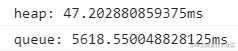### 什么是堆？

`[37, 22, 26, 12, 11, 25, 7, 3, 8, 10]`，初看起来这是一个数组，但如果我们把它按照二叉树的结构排列起来，就会出现图下的结构：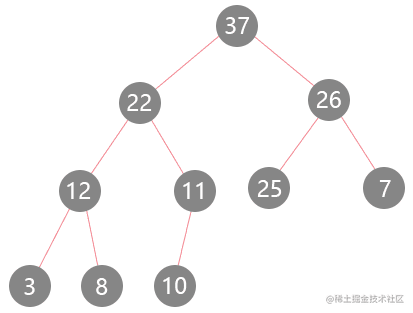1. 父节点优先级高于或等于子节点

2. 完全二叉树

3. 动态性

### 从零实现最大堆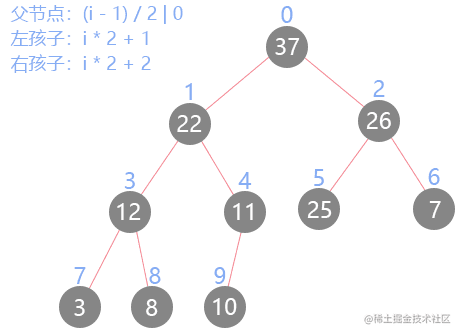``````class MaxHeap {
constructor() {
this._data = [] // 存储堆里的数据
}
getParent(i) { // 获取父节点
return (i - 1) / 2 | 0 // 向下取整
}
getLeftChild(i) { // 获取左孩子
return i * 2 + 1
}
getRightChild(i) { // 获取右孩子
return i * 2 + 2
}
}

#### 增(siftUp)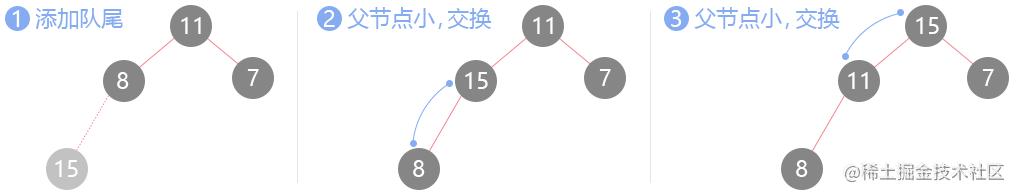``````class MaxHeap {
constructor() {
this._data = [] // 存储堆里的数据
}
...

push(val) {
this._data.push(val) // 添加到队尾
this.siftUp(this._data.length - 1) // 上浮队尾元素
}
siftUp(i) {
const parent = this.getParent(i) // 获取父节点的值
if (this._data[i] > this._data[parent]) { // 如果大于父节点的值
this.swap(i, parent) // 交换位置
this.siftUp(parent) // 继续上浮
}
}
swap(i, j) { // 交换数组两个元素的位置
[this._data[i], this._data[j]] = [this._data[j], this._data[i]]
}
}

#### 取(siftDown)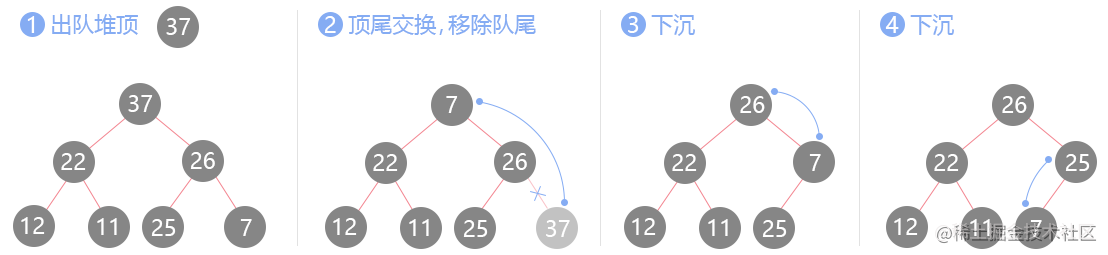``````class MaxHeap {
constructor() {
this._data = [] // 存储堆里的数据
}
...

sift() { // 出队
if (this._data.length === 0) {
return
}
const max = this._data // 缓存出队元素
this.swap(0, this._data.length - 1) // 顶尾交换
this._data.pop() // 移除队尾
this.siftDown(0) // 将换上来的节点进行下沉
return max // 返回应该出队的元素
}

siftDown() {
const { _data } = this
let max = this.getLeftChild(i) // 假如左孩子大
if (max >= _data.length) { // 左孩子界限超出，递归终止
return
}
if (max + 1 < _data.length && _data[max + 1] > _data[max]) {
// 如果有右孩子且右孩子比左孩子大，max+1变为右孩子的下标
max++
}
if (_data[i] < _data[max]) { // 如果孩子节点大于当前节点，执行下沉操作
this.swap(i, max) // 交换当前节点与孩子节点的位置
this.siftDown(max) // 在孩子节点的位置继续递归
}
}
}

#### 替换(replace)

``````class MaxHeap {
constructor() {
this._data = [] // 存储堆里的数据
}
...

replace(val) {
if (this._data.length === 0) {
return
}
const max = this._data // 缓存出队元素
this._data = val // 将添加的元素赋值给堆顶
this.siftDown(0) // 堆顶执行下沉操作
return max // 出队
}
}

#### 数组快速堆化(heapify)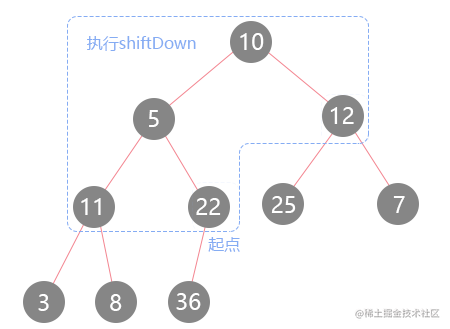``````class MaxHeap {
constructor(arr = []) { // 构造函数支持传入数组，快速堆化
if (Array.isArray(arr) && arr.length > 0) {
this._data = [...arr]
this.heapify(this._data)
} else {
this._data = [] // 存储堆里的数据
}
}
...

heapify(arr) {
let parent = this.getParent(arr.length - 1)
while (parent >= 0) {
this.siftDown(parent)
parent--
}
}
}

### 堆的应用

#### 堆排序 ↓

``````function heapSort(arr) {
let last = ((arr.length - 2) / 2) | 0; // 只需要从最后一个叶子的父节点开始
const ret = [];
while (last >= 0) {
siftDown(arr, last, arr.length); // 下沉一半的节点实现堆化
last--;
}
while (arr.length > 0) {
ret.push(arr); // 将堆顶元素放入新数组内
[arr, arr[arr.length - 1]] = [arr[arr.length - 1], arr]; // 交换首尾的元素
arr.pop(); // 将交换的堆顶元素去掉
siftDown(arr, 0, arr.length); // 重新下沉堆顶，不破坏堆的性质
}
return ret; // 返回排序好的数组

function siftDown(arr, i, n) {
let left = i * 2 + 1;
if (left >= n) {
return;
}
if (left + 1 < n && arr[left] > arr[left + 1]) { // 改为判断谁小谁 +1
left++;
}
if (arr[left] < arr[i]) { // 让大元素下沉
[arr[left], arr[i]] = [arr[i], arr[left]];
siftDown(arr, left, n);
}
}
}

#### 原地堆排序 ↓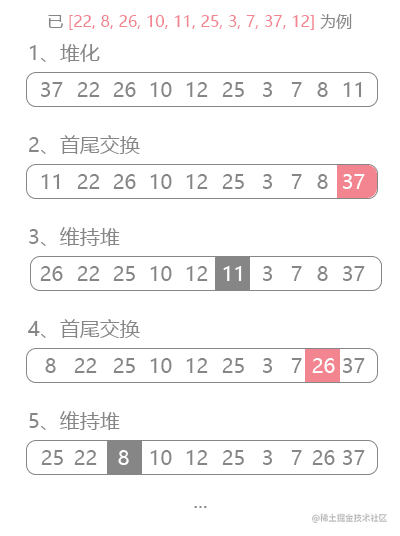``````function heapSort(arr) {
let last = ((arr.length - 1) / 2) | 0;
while (last >= 0) {
siftDown(arr, last, arr.length); // 使用最大堆
last--;
}
let r = arr.length;
while (r > 0) {
[arr, arr[r - 1]] = [arr[r - 1], arr]; // 将堆顶与末尾元素交换
r--; // 右边界减1
siftDown(arr, 0, r); // 重新下沉堆顶元素
}

function siftDown (arr, i, n) {
let left = i * 2 + 1;
if (left >= n) {
return;
}
if (left + 1 < n && arr[left] < arr[left + 1]) { // 大顶堆
left++;
}
if (arr[i] < arr[left]) { // 下沉小元素
[arr[i], arr[left]] = [arr[left], arr[i]];
siftDown(arr, left, n);
}
};
}

#### 1046-最后一块石头的重量 ↓

``````有一堆石头，每块石头的重量都是正整数。

`````` const lastStoneWeight = function (stones) {
let last = ((stones.length - 2) / 2) | 0;

while (last >= 0) { // 堆化
siftDown(stones, last);
last--;
}

while (stones.length > 1) { // 当只有一个元素结束循环
swap(stones, 0, stones.length - 1) // 首尾交换
const first = stones.pop() // 取出尾巴里的最大值
siftDown(stones, 0) // 执行下沉维持堆
const second = stones // 再取出堆顶的元素，即为第二大的元素
const diff = Math.abs(first - second) // 求出绝对值
stones = diff // 将绝对值覆盖堆顶
siftDown(stones, 0) // 再次维持堆
}
return stones;

function swap(arr, i, j) {
[arr[i], arr[j]] = [arr[j], arr[i]]
}

function siftDown(arr, i) {
let left = i * 2 + 1;
if (left >= arr.length) {
return;
}
if (left + 1 < arr.length && arr[left] < arr[left + 1]) {
left++;
}
if (arr[left] > arr[i]) {
swap(arr, left, i)
siftDown(arr, left);
}
}
};

#### 215-数组中的第K个最大元素 ↓

``````在未排序的数组中找到第 k 个最大的元素。

``````var findKthLargest = function (nums, k) {
const minHeap = [] // 最小堆
for (let i = 0; i < nums.length; i++) {
if (i < k) { // 堆的大小还没达到k
minHeap.push(nums[i]) // 直接push
siftUp(minHeap, minHeap.length - 1) // 执行上浮操作维持堆的性质
} else if (nums[i] > minHeap) { // 如果下一个元素大于堆顶
minHeap = nums[i] // 替换堆顶元素
siftDown(minHeap, 0) // 执行下沉操作维持堆性质
}
}
return minHeap // 最后返回最小堆堆顶元素即可
};

function siftUp(nums, i) {
const parent = (i - 1) / 2 | 0
if (nums[i] < nums[parent]) { // 将小的元素上浮
swap(nums, i, parent)
siftUp(nums, parent)
}
}

function siftDown(nums, i) {
let l = i * 2 + 1
if (l + 1 > nums.length) {
return
}
if (l + 1 <= nums.length && nums[l] > nums[l + 1]) {
l++  // 选取小的元素
}
if (nums[i] > nums[l]) { // 将大的元素下沉
swap(nums, i, l)
siftDown(nums, l)
}
}

function swap(nums, i, parent) {
[nums[i], nums[parent]] = [nums[parent], nums[i]]
}

#### 373-查找和最小的K对数字 ↓

``````给定两个以升序排列的整形数组 nums1 和 nums2, 以及一个整数 k。

[1,2],[1,4],[1,6],[7,2],[7,4],[11,2],[7,6],[11,4],[11,6]

``````var kSmallestPairs = function (nums1, nums2, k) {
const heap = []
for (let i = 0; i < nums1.length; i++) {
for (let j = 0; j < nums2.length; j++) {
if (heap.length === k && nums1[i] + nums2[j] >= (heap + heap)) {
// 因为都是升序的数组，所以当这次的和大于堆顶时，
// 那么之后的循环也一定是大于的，所以跳出这轮循环即可。
break
}
if (heap.length < k) { // 堆没有满时
heap.push([nums1[i], nums2[j]])
siftUp(heap, heap.length - 1) // 构建最大堆
} else if ((nums1[i] + nums2[j]) <= sum(heap)) { // 只有当小于堆顶时
heap = [nums1[i], nums2[j]] // 替换堆顶的元素
siftDown(heap, 0) // 维持最大堆
}
}
}
return heap.sort((a, b) => (a + a) - (b + b))
// 因为是最大堆，所有返回升序的顺序
};

function siftUp(heap, i) {
const parent = (i - 1) / 2 | 0
if (sum(heap[i]) > sum(heap[parent])) {
swap(heap, i, parent)
siftUp(heap, parent)
}
}

function siftDown(heap, i) {
let left = i * 2 + 1
if (left >= heap.length) {
return
}
if (left + 1 < heap.length && sum(heap[left]) < sum(heap[left + 1])) {
left++
}
if (sum(heap[i]) <= sum(heap[left])) {
swap(heap, i, left)
siftDown(heap, left)
}
}

function sum(arr) { // 求和
return arr + arr
}

function swap(arr, i, j) {
[arr[i], arr[j]] = [arr[j], arr[i]]
}